×#### Thank you for registering.

One of our academic counsellors will contact you within 1 working day.

Click to Chat

1800-1023-196

+91-120-4616500

CART 0

• 0

MY CART (5)

Use Coupon: CART20 and get 20% off on all online Study Material

ITEM
DETAILS
MRP
DISCOUNT
FINAL PRICE
Total Price: Rs.

There are no items in this cart.
Continue Shopping• Complete JEE Main/Advanced Course and Test Series
• OFFERED PRICE: Rs. 15,900
• View Details

```Chapter 15: Linear Inequations – Exercise 15.4

Linear Inequations – Exercise – 15.4 – Q.1

Let x be the smaller of the two consecutive odd positive intgers. Then the other odd integer is x + 2.

It is given that both the integers are smaller than 10 and their sum is more than 11.

∴ x + 2 < 10 and, x + (x + 2) > 11

⟹ x < 10 - 2 and 2x + 2 > 11

⟹ x < 8 and 2x > 9

⟹ x < 8 and x > 9/2

⟹ 9/2 < x < 8

⟹ x = 5, 7         [ ∵ x is an odd integer]

Hence, the reuired pairs of odd integers are (5, 7)  and (7, 9).

Linear Inequations – Exercise – 15.4 – Q.2

Let x be the smaller of the two consecutive odd natural numbers. Then the other odd integer is x + 2.

It is given that both the natural number are greater than 10 and their sum is less than 40.

∴ x > 10 and, x + x + 2 < 40

⟹ x > 10 and 2x < 38

⟹ x > 10 and x < 19

⟹ 10 < x < 19

⟹ x = 11, 13, 15, 17                     [͙∵ x is an odd number]

Hence, the required pairs of odd natural number are (11, 13), (13, 15), (15, 17) and (17,19).

Linear Inequations – Exercise – 15.4 – Q.3

Let x be the smaller of the two consecutive even positive integers.

Then the other even integer is x + 2.

It is given that both the even integers are greater than 5 and their sum is less than 23.

∴ x > 5 and, x + x + 2 < 23

⟹ x > 5 and 2x < 21

⟹x > 5 and x < 21/2

⟹ 5 < x < 21/2 = 10.5

⟹ x = 6, 8, 10   [∵ x is an even integer]

Hence, the required pairs of even positive integer are (6, 8), (8, 10) and (10, 12).

Linear Inequations – Exercise – 15.4 – Q.4

Suppose Rohit scores x marks in the third test then,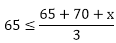⟹ 195 ≤ 135 + x

⟹ 195 – 135 ≤ x

⟹ 60 ≤ x

Hence, the minimum marks rohit should score in the thrid tes is 60.

Linear Inequations – Exercise – 15.4 – Q.5

We have,

F1 = 86°F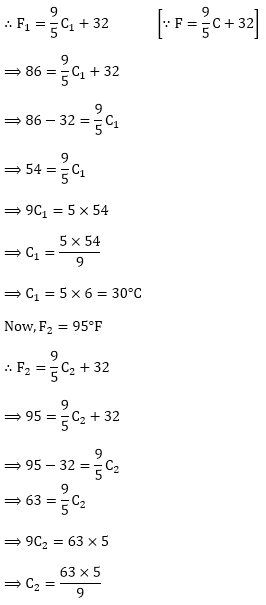⟹ C2 = 7 × 5 = 35°C

∴ The range of temperature of the solution is from 30°C to 35°C.

Linear Inequations – Exercise – 15.4 – Q.6

We have,

C1 = 30°C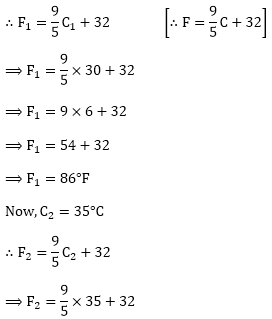⟹ F2 = 9 × 7 + 32

⟹ F2 = 63 + 32

⟹ F2 = 95°F

∴ Hence, the temperature of the solution lies between 86°F to 95°F.

Linear Inequations – Exercise – 15.4 – Q.7

Suppose Shikha scores x marks in the fifth paper. Then,⟹ 90 × 5 ≤ 182 + 186 + x

⟹ 450 ≤ 368 + x

⟹ 450 - 368 ≤ x

⟹ 82 ≤ x
Hence, the minimum marks is required in the last paper is 82.

Linear Inequations – Exercise – 15.4 – Q.8

We have,

Profit = Revenue - cost

Therefore, to earn some profit, we must have

Revenue > Cost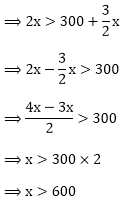Hence, the manufacturer must sell more than 600 cassettes to realize some profit.

Linear Inequations – Exercise – 15.4 – Q.9

Let the length of the shortest side be x.

Then, the length of the longest side and third side of the triangle are 3x and 3x - 2 respectively.

According to question,

Perimeter of triangle ≥ 61

⟹ x + 3x – 2 + 3 x ≥ 61

⟹ 7x ≥ 61 + 2

⟹ 7x ≥ 63

⟹ x ≥ 63/7

⟹ x ≥ 9

∴ The minimum length of the shortest side is 9 cm.

Linear Inequations – Exercise – 15.4 – Q.10

Let the quantity of water to be added to solution = x liters.

∴ 25 % (1125 + x) < 45% of 1125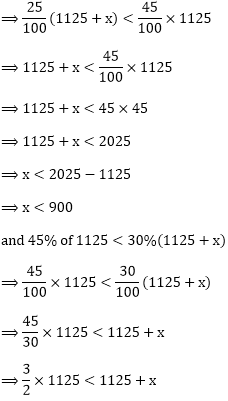⟹ 1.5 × 1125 < 1125 + x

⟹ 1687.5 < 1125 + x

⟹ 1687.5 – 1125 < x

⟹ 562.5 < x            ………  (ii)

Using (i) and (ii), we get 562.5 < x < 900

Hence, quantity of water lies between 562.5 litres and 900 litres.

Linear Inequations – Exercise – 15.4 – Q.11

Let x liters of 2% solution will have to be added to 640 liters of the 8% solution of acid.

Total quantity of mixture = (640 + x)

Total acid in the (640 + x) liters of mixture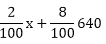It is given that acid content in the resulting mixture must be more than 4% but less than 6%.⟹ 4[640 + x] < (2x + 8640) < 6[640 + x]

⟹ 2560 + 4x < 2x + 8640 and 2x + 8640 < 3840 + 6x

⟹ 2560 – 8640 < 2x - 4x and 2x - 6x < 3840 - 8640

⟹ x < 1280 and x > 320

More than 320 litres but less than 1280 liters of 2% is to be added.

```### Course Features

• 728 Video Lectures
• Revision Notes
• Previous Year Papers
• Mind Map
• Study Planner
• NCERT Solutions
• Discussion Forum
• Test paper with Video Solution